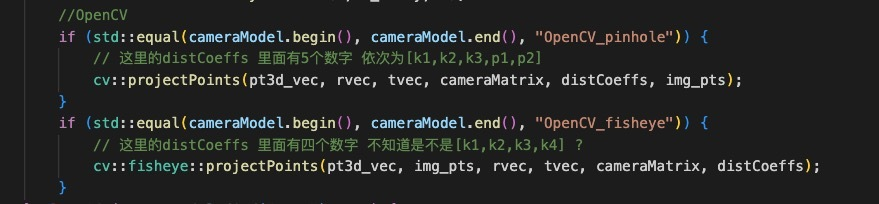﻿ 技术分享

# 关于3d场景坐标映射对应2d相机的畸变处理实践

https://zhuanlan.zhihu.com/p/94445955

1.根据相机矩阵转换为相机坐标(x相,y相,z相) z轴正方向为相机朝向,x轴向右,y轴向下
2.坐标归一化得到 (x归,y归,1) = (x相/z相,y相/z相,1)
3.得到距离 r = sqrt(x归^2+y归^2)
4.这时候进行相机畸变调整 得到x',y'

``````x' = x归*(1+k1*r^2+k2*r^4+k3*r^6)+2*p1*x归*y归+p2*(r^2+2*x归^2)
y' = y归*(1+k1*r^2+k2*r^4+k3*r^6)+2*p2*x归*y归+p1*(r^2+2*y归^2)``````

5.(x',y',1)乘以相机内参得到最终(u,v)值https://zhuanlan.zhihu.com/p/577186117
https://blog.csdn.net/qq_39642978/article/details/112758040

(x',y',1) 乘以相机内参得到图片像素(u,v)

https://blog.csdn.net/qq_16137569/article/details/112398976

﻿